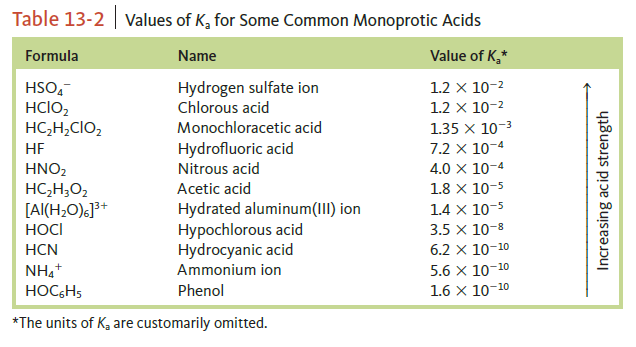# Problem: Using the Ka values in the following Table, calculate the percent dissociation in a 0.20-M solution of the following acid.c. phenol (HOC6H5)

###### FREE Expert Solution
91% (212 ratings)
###### Problem Details

Using the Ka values in the following Table, calculate the percent dissociation in a 0.20-M solution of the following acid.

c. phenol (HOC6H5)